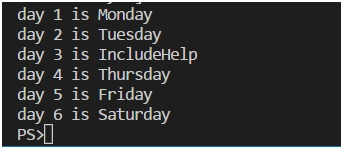# Loops in JavaScript

In this article, we are going to learn various types of looping statements in JavaScript with syntax, example and explanation.
Submitted by Himanshu Bhatt, on August 09, 2018

Loops? Well like the name suggests, these are the block which repeats itself when some certain conditions are met. So, we will discuss here some day to day most useful loops.

### The For Loop

For loop have the following format for(initialization, condition, updation) now, what it means? We see an example here:

```for(let i = 0; i<10; i++){
console.log(i);
}
```

Now we observed that the let i = 0 is a part of initialization that means i will be declared for this for block. i < 10 is the condition here so whenever i will be lesser than 10 the for block will run, and last i++ simply a shorthand for i = i + 1 that is variable i will increase by one every time it completes the block.

### The While Loop

Just like for loop, while loop is also a most useful loop. The format for while loop is while(condition).

Let’s see an example,

```let i = 10;
while(i>0){
console.log(i);
i--; //line 1
}
```

Here, we already have a variable before while loop, and it only needs one condition, in this case, i must be greater than 10. Now in line 1 we used i-- which is a shorthand for i = i – 1 and like you can notice we make an update by ourselves in itself the while loop and it’ll run till the condition is true.

Now we begin one of the most useful methods for the arrays, that is, 'forEach()'

This forEach method is like any other loop whether a for loop or a while loop.

```week.forEach(function(days,index){
console.log('day \${index+1} is',days);
})
```

forEach performs the specified action for each element in an array. The forEach method accepts 2, arguments first a callback function and another thisArg.

Callback function: Well we can call a function after declaring it as an argument of forEach(). But a callback function is what we declare at the time of calling. When we use a callback function in forEach we can use at most 3 arguments, first for values/elements of the array and second for index and last one as a string.

thisArg: An object to which this keyword can refer in the callback function. If thisArg is omitted, undefined is used as this value.

forEach() method will call the callback function for each element of the array, the days (in above example) will hold one element at a time and index will hold the index for the corresponding element.

Thus, from the above code, we have the following output:To understand more what are the callback functions, read: Understanding callbacks in JavaScript